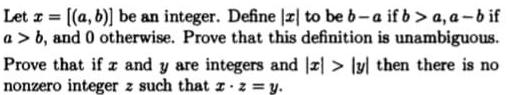Question:

# Let z = [(a, b)] be an integer. Define [z] to be b-a if b>a,

Last updated: 7/4/2022Let z = [(a, b)] be an integer. Define [z] to be b-a if b>a, a-b if a> b, and 0 otherwise. Prove that this definition is unambiguous. Prove that if z and y are integers and z> [y] then there is no nonzero integer z such that x.z = y.